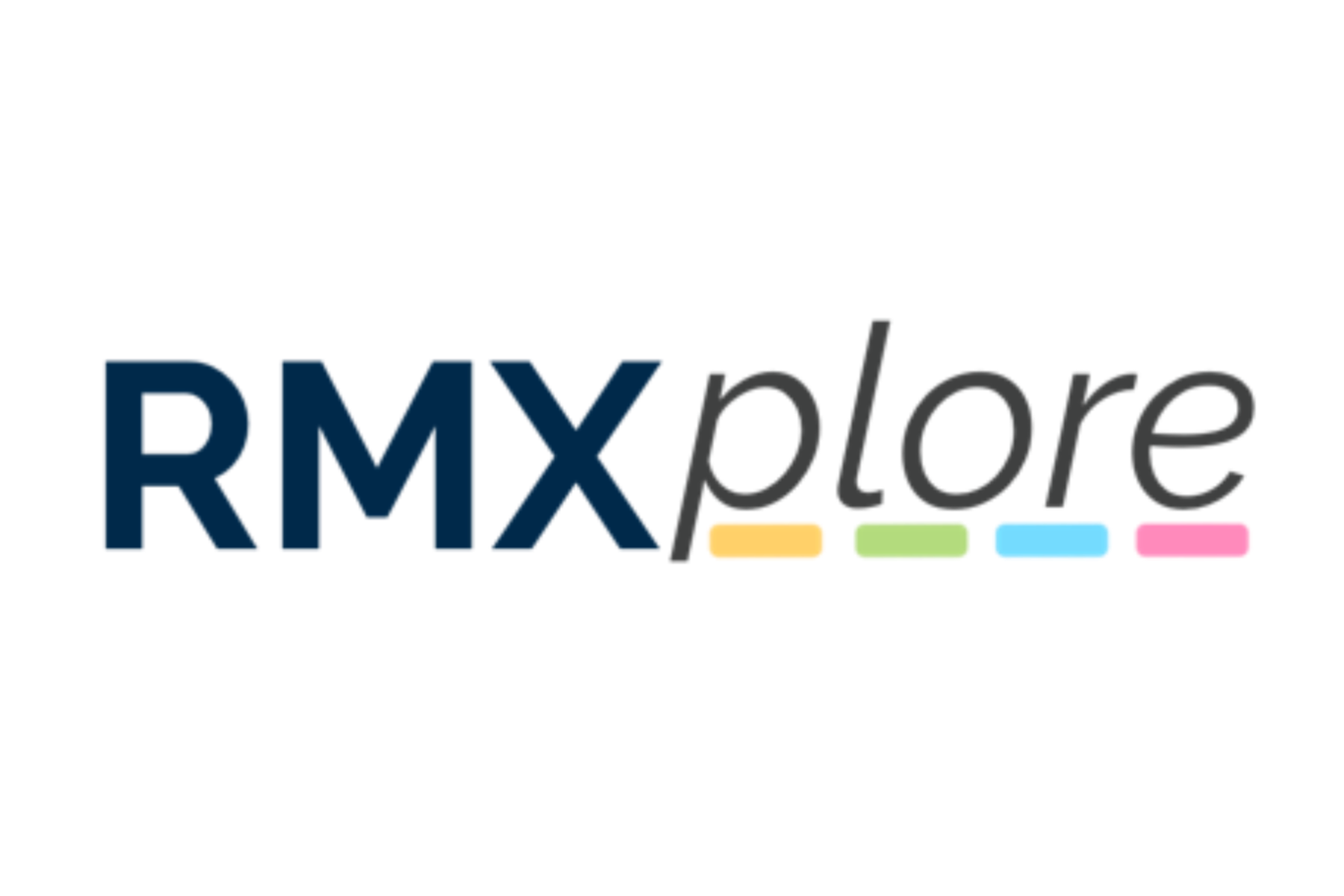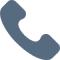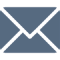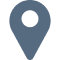# Operations, Measurement, and Thinking (OMT) II

Please note that RMXplore's Math Bytes Courses are not
currently open for registration
.

### Operations, Measurement, and Thinking (OMT) II

Coming Soon!
Course Pricing:
• Online : \$260
• Houston Center : \$320
• Cupertino Center : \$400
Course Logistics:
Duration : to be updated
Number of Classes: 5 weeks course
Occurs : to be updated

### Math Byte Course Topics:

Course Topics are organized as six modules with five granular topics covered under each module. Click through below to see all modules/topics for this course.

#### 01 - Additions and Subtraction• 1-digit multiplication tables
• Multiplication within tables
• Long division with remainders
• Multiplication and division up to 100
• Multiplication/division of 3 digit by 1 digit

#### 02 - Multiplication and Division• 1-digit multiplication tables
• Multiplication within tables
• Long division with remainders
• Multiplication and division up to 100
• Multiplication/division of 3 digit by 1 digit

#### 03 - Fractions• Interpreting a fraction
• Comparing and ordering like fractions
• Addition and subtraction of like fractions
• Listing equivalent fractions
• Simplifying, comparing and ordering unlike fractions

#### 04 - Measurement• Measure lines of a given length, estimating distance
• Measure volume and masses
• Unit conversions between kg/g, l/ml, m/cm, etc.
• Counting money
• Interpreting the minute hand

#### 05 - Elementary Geometry• Semicircle, quarter circle
• Introduction to lines, curves, and faces
• Area and perimeter of squares and rectangles
• Parallel, perpendicular lines
• Angles: Acute, obtuse, right angles

#### 06 - Word Problems